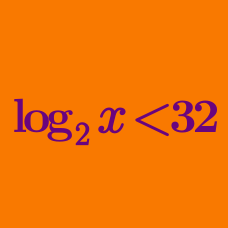Algebra

# Logarithmic Inequalities - Similar Base

Solve the logarithmic inequality $\log_{25}\left(3 x^2+4x+1\right)\le \log_{5}(x+1)+1.$

Solve $\log_2(x-13)<\log_4(x-10)+1.$

How many integers $$x$$ satisfy the logarithmic inequality $\log_{2}(x-1)\ge \log_{8}(2x^3-13x^2+20x-9)?$

How many integer solutions does the following inequality have: $\log_{3}(x-1)\le \log_{9}(313-x^2)?$

What is the minimum integer $$x$$ that satisfies the inequality $\log_{2}(x-4)>\log_{4}(x-2)?$

×

Problem Loading...

Note Loading...

Set Loading...